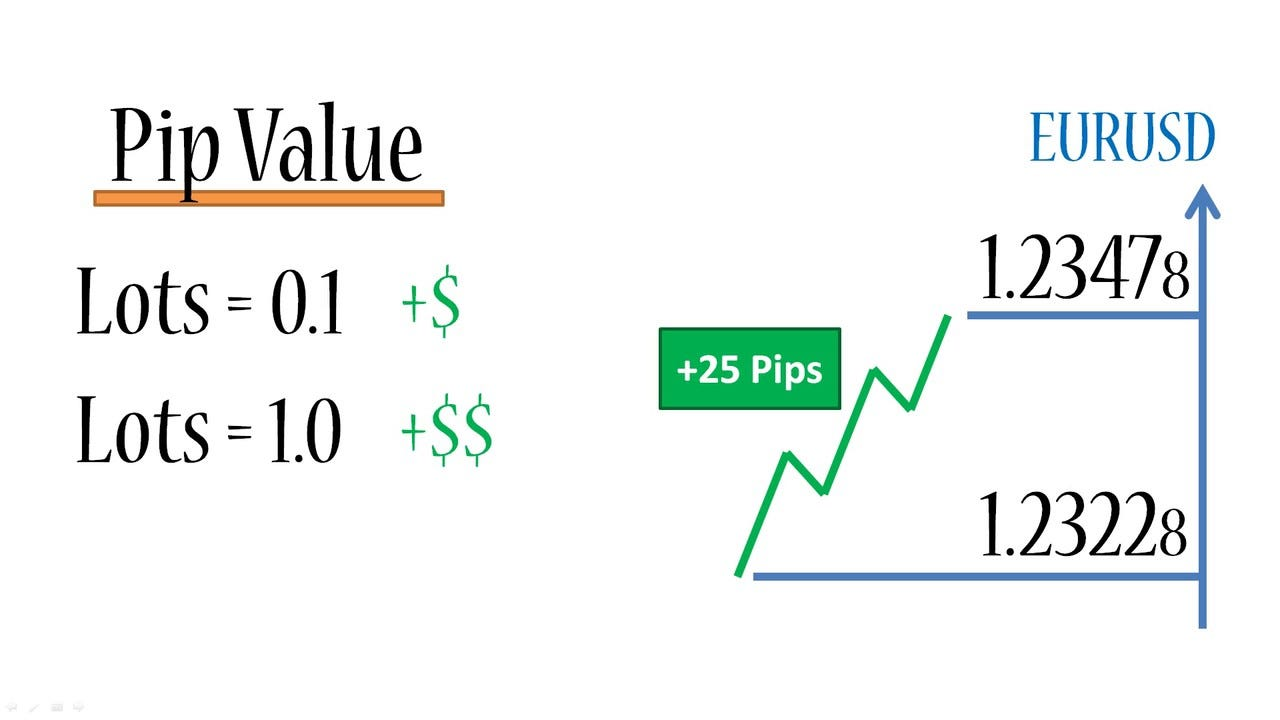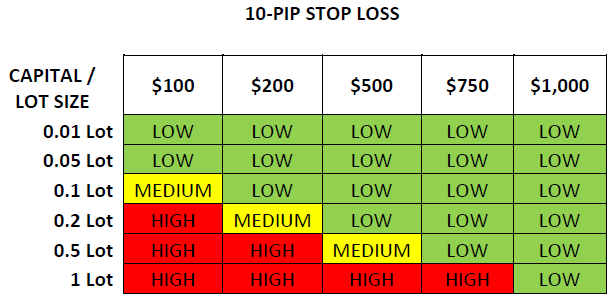Home Beginners Forex Education Forex Basics Pips and Lots: What They Are & How They Differ

# Pips and Lots: What They Are & How They Differ

474
0In this article, we have to do some basic math. It is very likely that you have heard the terms “lot” and “pips” and if you’ve read about the Forex market. Below we will show you what they are and how they are calculated.

Take the time to digest this information, as it is vital knowledge that every Forex investor must learn and handle. Don’t even think about starting trading in Forex without being able to calculate the value of a pip and without being able to calculate gains and losses.

#### What is a Pip?

A pip is the smallest possible change in the value of a currency pair. If for example the EUR/USD pair moves from 1.3150 to 1.3151, that is 1 PIP. A pip is the last decimal place in the quotation. Through the pips, you will calculate the gains and losses. As each currency kept a value, is appropriate to calculate the value of a pip for each particular currency.

In pairs where the US dollar (USD) is the base currency, the calculation would be as follows:

Imagine the USD/JPY pair at a value of 119.80 (you will see that for this pair only two decimals are used, while the vast majority use four decimals).

For USD/JPY, 1 pip equals .01

By this, we mean:

USD/JPY:

119.80

.01 divided by quotation = value of a pip.

.01 / 119.80 = 0.0000834

May appear to be too small a number, but then we’ll see how everything is relative to the size of the lot.

USD/CHF:

1,5250

.0001 divided by quotation = value of pip.

.0001 / 1.5250 = 0.0000655

1,4890

.0001 divided by quotation = value of pip.

.0001 / 1.4890 = 0.00006715

In the case where the dollar (USD) is not the base currency, and we want to get the dollar value of a pip, an additional step will be required.

EUR/USD:

2200

.0001 divided by quotation = value of a pip.

Thus

.0001 / 1.2200 = EUR 0.00008176But we want to know the value of the dollar, so do one more calculation…

EUR x Quote

Thus

0.00008196 x 1.2200 = 0.00009999

We’ll round it up to 0,0001.

GBP/USD

1.7975

.0001 divided by quotation = value of pip.

Thus

.0001 / 1.7965 = GBP 0.0000556

But we want to know the value of the dollar, so we do one more calculation…

GBP x Quote.

Thus

.0000556 x 1.7975 = 0.0000998

We rounded it up to 0,0001.

In the next section, we will find out how these numbers that might seem insignificant can have a big impact when investing in Forex.#### What is One Batch?

In Forex it is operated in batches. The standard size of a batch is \$100,000. There are also mini-batches that are \$10,000. And there are even micro-lots of \$1,000. As you’ve already learned, currencies are measured in pips, which are the minimum possible increase. To get any benefit out of these small increases, we need to trade large amounts of a particular currency in order to achieve any significant gain or loss.

Let’s assume we’re going to use a standard batch of \$100,000. We’ll do some calculations to see how the value of a pip is affected.

USD/JPY at a rate of 119.90

(.01 / 119.80) x \$100,000 = \$8.343 per pip

USD/CHF at a rate of 1.4556

(.0001 / 1.4556) x \$100,000 = \$6.87 per pip

In the case where the dollar is first, the formula changes a little.

EUR/USD at a rate of 1.1920

(.0001 / 1.1920) x EUR 100K = EUR 8,38 x 1.1920 = \$9.99735 and rounded to \$10 per 1 pip.

GBP/USD at a rate of 1.8045

(.0001 / 1.8045) x GBP 100K

= 5.54 x 1.8045 = 9.99416 and rounded to \$10 per 1 pip.

Depending on the online broker we work with, they may have some different particularities when calculating the value of a pip relative to the size of a lot. But in any case, as long as market prices vary, so can the value of a pip vary according to the currency being used.

#### How Do I Calculate Profits and Losses?

We already know how to calculate the value of a pip, then let’s see how we can calculate our profits or losses.

Let’s take an example where we buy US dollars (USD) and sell Swiss Francs (CHF). Let’s imagine that the quote is at 1.4525/1.4530. As we are buying USD, we use the price of the ask, which is 1.4530. We bought 1 lot of \$100,000 to 1,4530. A few hours later, the price went up to 1.4550 and it was decided to close the deal.

The new rate is 1,4545 /1,4550. Since we are closing the transaction and initially made a purchase to start the operation, we need to close the same transaction with a sale, with a price of 1.4545. The difference between 1.4520 and 1.4540 is .0020 or 20 pips.

Using our formula above, we calculated a gain of (.0001/1.4550) x \$100,000 = \$6.86 per pip x 20 pips = \$137.40.

Remember that when you open a position, you are subject to the spread which is the difference between the bid/ask and is the commission that the brokers receive for executing the transaction. When buying, the ask price will be used, and when selling the bid price will be used.#### What is Leverage?

We’ve already talked a little bit about leverage in the previous article (How do you make money in Forex?) but if you haven’t seen it yet, you’re probably wondering how a small investor like yourself could handle such large sums of money. Think of your Forex broker as a bank that lends you \$100,000 to buy currencies but only asks for a \$1000 deposit as a good-faith guarantee or guarantee to perform the transaction. This sounds too nice to be true, but that’s how leverage is used in the Forex currency market or in some other investment instruments.

The level of maximum leverage available to use depends on the broker you work with and can several from one investment instrument to another. Online brokers offering services to retail customers generally require a very small minimum initial deposit to open a trading account. Once you have deposited that money, you can trade on Forex. The broker will also tell you what margin you need to have available in your account as a guarantee to perform operations

For example, imagine that your broker offers you a leverage of 1:100. For every \$1000 you have available in your account, you can open operations for 1 batch of \$100,000. So if you have \$5,000, you could manage a position of \$500,000 (5 lots).

The margin for each lot (margin) may vary considerably from one broker to another. In the above example, the broker requires a margin of 1%. This means that for every \$100,000 invested, the broker occupies a \$1000 deposit as collateral.

#### What is a Margin Call?

In addition to the guarantee margin required to open a position, there is also a maintenance margin to keep your position open. In the event that the money in your trading account falls below the required margin requirements, the broker will close some of the positions you have open to put your balance sheet and account back within the required margin. This is a measure to prevent you from having a negative balance sheet and incurring debt. These measures to avoid negative balances are executed automatically according to the evolution of your positions, even in a highly volatile and fast environment like that of the Forex market.

Example #1

Suppose you open an account with \$2000 and buy a lot of EUR/USD with a margin requirement of \$1000. The margin you can use is the capital available to start new positions or manage losses. As started with \$2000, the usable margin is \$2000. But when you open a lot, which requires a \$1000 margin, the margin available will now be \$1000.

If your position goes into losses and those \$1000 that remain free in your trading account do not cover the maintenance margin requirements the margin call or margin call will occur.

Example #2

Suppose you open a \$10,000 Forex account. You trade 1 batch of EUR/USD, with a \$1000 margin requirement. Remember that the available margin can be used to open new positions or to sustain the eventual losses of current open positions. Before opening the position, you would have a \$10,000 margin available. Once you open the position, you have a \$9000 usable margin.

Make sure you understand the difference between usable margin and the margin used. If your account balance falls below the usable margin due to losses, you will need to deposit more money or the broker will proceed to close the position to limit the risk to both you and them. As a result of this, you can never lose more than the amount you have deposited. It is vital to know the requirements regarding the online broker margin you will use and also feel comfortable with the risk you are taking in each transaction.